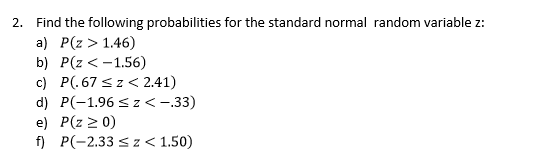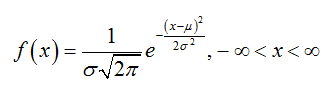# Find the following probabilities for the standard normal random variable za) P(z > 1.46)b) P(z

Question
541 views

Part a, b, and chelp_outlineImage TranscriptioncloseFind the following probabilities for the standard normal random variable z a) P(z > 1.46) b) P(z <-1.56) c) P(.67 s z<2.41) d) P(-1.96 sz< -.33) e) P(z 2 0) f P(-2.33 z<1.50) 2. fullscreen
check_circle

Step 1

Normal distribution:

A continuous random variable X is said to follow normal distribution with mean (m) and standard deviation (σ) if the probability density function of X is,Step 2

Standard normal distribution:

The standard normal distribution is a special case of normal distribution. The standard normal distribution will have mean 0 and standard deviation 1. If a random variable X follows normal distribution with mean (m) and standard deviation (σ), then the standard normal variable z will be as given below:

Step 3

An important property of the normal distribution:

The normal distribution is symmetric. Thus, for any pai...

### Want to see the full answer?

See Solution

#### Want to see this answer and more?

Solutions are written by subject experts who are available 24/7. Questions are typically answered within 1 hour.*

See Solution
*Response times may vary by subject and question.
Tagged in

### Other